Impact Factor 5.221 | CiteScore 4.1
More on impact ›

# Frontiers in Chemistry## REVIEW article

Front. Chem., 26 October 2018 | https://doi.org/10.3389/fchem.2018.00503

# Wavelet Transform-Based UV Spectroscopy for Pharmaceutical Analysis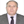Erdal Dinç1* andZehra Yazan2
• 1Department of Analytical Chemistry, Faculty of Pharmacy, Ankara University, Ankara, Turkey
• 2Department of Chemistry, Ankara University Faculty of Science, Ankara, Turkey

In research and development laboratories, chemical or pharmaceutical analysis has been carried out by evaluating sample signals obtained from instruments. However, the qualitative and quantitative determination based on raw signals may not be always possible due to sample complexity. In such cases, there is a need for powerful signal processing methodologies that can effectively process raw signals to get correct results. Wavelet transform is one of the most indispensable and popular signal processing methods currently used for noise removal, background correction, differentiation, data smoothing and filtering, data compression and separation of overlapping signals etc. This review article describes the theoretical aspects of wavelet transform (i.e., discrete, continuous and fractional) and its characteristic applications in UV spectroscopic analysis of pharmaceuticals.

## Introduction

In experimental studies, instruments or devices can provide signals (or graphs) in different formats e.g., spectrum, chromatogram, voltammogram, and electroferogram etc. The analysis of chemicals and pharmaceuticals in various samples is based upon the utilization of the measured signals of substances of interest. In practice, such an analysis for a multicomponent mixture may not be determined without a prior separation step due to spectral overlapping. Therefore, high performance liquid chromatography (HPLC) is one of the most commonly used techniques for quantitative estimation in the quality control of raw materials and commercial products in laboratories. In some cases, chromatographic determination could not be possible due to not only similar physicochemical behavior of analytes but also time- and solvent-consumption for optimal experimental conditions.

In practice, UV spectroscopic methods are widely used in chemical and pharmaceutical analysis. As compared to chromatographic ones, the use of spectroscopic methods provides a rapid analysis with low-cost and acceptable results. However, multicomponent analysis may not be possible with a traditional UV spectrophotometric approach due to spectral interferences of both active and inactive ingredients in samples. In some cases, derivative spectrophotometry (O'Haver and Green, 1976; O'Haver, 1979; Levillain and Fompeydie, 1986; Ragno et al., 2006) and its improved versions e.g., ratio spectra-derivative spectrophotometry (Salinas et al., 1990), ratio spectra-derivative spectrophotometry-zero crossing (Berzas Nevado et al., 1992; Dinç and Onur, 1998; Dinç, 1999), and double-divisior-ratio spectra-derivative spectrophotometry (Dinç and Onur, 1998; Dinç, 1999; Gohel et al., 2014; Shokry et al., 2014) could be used in place of conventional UV spectrophotometric method for analysis of binary and ternary mixtures without using a separation step. However, these spectral approaches may not always yield successful data due to severely overlapping spectral bands, spectral noise and baseline variation. Additionally, high-order differentiation of spectra may lead to spectral deterioration i.e., a decrease in signal intensity and signal-to-noise ratio. As a result, a number of mathematical manipulations (or signal processing methods) are often required to make instrumental signals more meaningful for analysis purpose.

Generally speaking, transform (i.e., Fourier, Hilbert, short-time Fourier, Wigner distribution, Radon, and wavelet) is a very suitable technique in the pre-treatment step to simplify signals. Fourier transform (FT) is the first method to modify chemical signal (Griffiths, 1977; Cooper, 1978; Griffiths and De Haseth, 1986; Ernst, 1989) with the mathematical essence such as filtering, convolution/deconvolution etc. FT analysis can localize signal in frequency domain very well, but not so much in time domain. In contrast, wavelet transform (WT) has the advantage of localizing signals both in time (position) and frequency (scale) domains, making it a preferable mathematical tool to replace FT in the study of the local property of a signal and the removal of the perturbation of measuring error in spectral analysis. Nowadays, WT is one of the most signal analysis algorithms commonly used in the different fields of chemistry and engineering, providing alternative ways or opportunities to resolve complex spectral bands or diverse data types of signals.

For readers interested in learning the general theory of wavelets, more details can be found in the literature (Mallat, 1988; Chui, 1992; Daubechies, 1992; Newland, 1993; Byrnes et al., 1994; Chui et al., 1994; Vetterli and Kovačević, 1995; Strang and Nguyen, 1996).

In the signal smoothing and de-noising of spectral peaks, the elimination of noise requires an application of appropriate filters to the raw spectral data such as some conventional signal filters Savitzky–Golay, Fourier and Kalman (Brown et al., 1994, 1996). The use of WT in signal analysis is two-fold: (i) to detect the singularities of a signal very likely caused by high-frequency noise and (ii) to separate the signal frequencies at different scales (Palavajjhala et al., 1994; Yan-Fang, 2013; Li and Chen, 2014). To illustrate this, Barclay et al. (1997) performed a comparative study in de-noising and smoothing of Gaussian peak by using wavelet, Fourier and Savitzky–Golay filters i.e., smoothing eliminates high-frequency components of the transformed signal irrespective of their amplitudes, while de-noising eliminates small-amplitude components of the transformed signal irrespective of their frequencies.

Historically, WT principal applications in chemistry were first explored by Walczak and Massart (1997a), who presented an approach based on the application of wavelet packet transform (WPT) to the best-basis selection for the compression and de-noising of a set of signals in time-frequency domain. In their paper, the proposed technique was compared to Wickerhauser's approach (Wickerhauser, 1994) of fast approximate principal component analysis (PCA). These authors also published two more papers on the application of wavelets for data processing i.e., the introduction of WPT for noise suppression and signal compression (Walczak and Massart, 1997b) and the use of WT for signal compression and denoising, image processing, data compression and multivariate data modeling in analytical chemistry (Walczak and Massart, 1997c). On the other hand, Alsberg et al. (1997) tried to introduce WT to chemometricians by suggesting the short-time FT technique as a resolution to obtain information about frequency changes over time as well as the WT for de-noising, baseline removal, determination of derivative zero crossings and signal compression. In 1997, WT application in chemical analysis was also confirmed by Wang et al. (1997) and Depczynski et al. (1997). Up to date, WT processing of the different types of raw signals has been reported for liquid chromatography (Shao et al., 1997, 1998a,b,c) and NMR spectroscopy (Neue, 1996; Barache et al., 1997), Raman spectra (Cai et al., 2001; Ehrentreich and Summchen, 2001), and voltammetry (Chen et al., 1996; Fang and Chen, 1997; Zheng et al., 1998; Zhong et al., 1998; Aballe et al., 1999; Zheng and Mo, 1999) IR and Raman spectroscopy (Shao and Zhuang, 2004; Hwang et al., 2005; Chalus et al., 2007; Jun-fang et al., 2007; Lai et al., 2011). In this context, as in the various fields of mathematics and engineering, the implementations of WT in analytical chemistry and neighbor disciplines has become increasingly attractive as an alternative way to analyze complex mixtures previously unresolved by traditional analytical techniques.

With reference to the above-mentioned review, the aim of this paper is to describe the fundamentals of WT methodologies and its typical implementations for UV spectroscopic analysis of pharmaceuticals.

## Brief History of Wavelets

In the literature, the first study was related to the Haar Wavelet transform. This family was suggested by the mathematician Alfred Haar in 1909. However, the word “wavelet” was not used in the period of Haar. In fact, the word “wavelet” was invented by Morlet and the physicist Alex Grossman in 1984. After the first orthogonal Haar wavelet, the second orthogonal wavelet known as “Meyer wavelet” was formulated by the mathematician Yves Meyer in 1985. In 1988, Stephane Mallat and Meyer elaborated the concept of multiresolution. In the same year, a systematical method to construct compactly supported continuous wavelets was found by Ingrid Daubechies. Afterwards, Mallat proposed the fast wavelet transform. The emergence of this algorithm increased the implementations of the WT in the signal processing field.

In other words, the history of the wavelet families could be given in the following chronological order: Haar families in 1910, Morlet wavelet concept in 1981, Morlet and Grossman, “wavelet” in 1984, Meyer, “orthogonal wavelet” in 1985, Mallat and Meyer, multiresolution analysis in 1988, Daubechies, compact support orthogonal wavelet in 1988 and Mallat, fast wavelet transform in 1989 (c.f. Chun-Lin, 2010).

Basically, WT can be mainly classified into discrete wavelet transform (DWT) and continuous wavelet transform (CWT) in the signal analysis. The theory and implementations of wavelets in chemistry and related fields were well documented as review papers (Leung et al., 1998; Dinç and Baleanu, 2007b; Dinç, 2013; Li and Chen, 2014; Medhat, 2015) and reference books (Walczak and Massart, 2000a,b; Walczak and Radomski, 2000; Brereton, 2003, 2008; Chau et al., 2004; Danzer, 2007; Mark and Workman, 2007; Dubrovkin, 2018).

## Wavelet Transform Algorithms

FT is based upon the decomposition of a signal into a set of trigonometric (sine and cosine) functions i.e., FT represents a signal in terms of sinusoids. The representation of FT of a signal from time mode to frequency mode is illustrated in Figure 1. For the determination of a local information in the FT, it is required to use an analyzing function ψ having localization properties in both frequency and time domains. This ψ function is named as a wavelet and it must be wave of finite duration.

FIGURE 1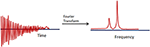Figure 1. Representation of Fourier transform of a signal from time domain to frequency domain.

WT contains the decomposition of a signal into a set of basic functions (wavelets). Basis functions of WT are small waves detected in different times. On the contrary to FT, WT gives information on both time and frequency, making it as an alternative approach to eliminate the resolution problem in signal analysis.

By definition, wavelets are the mathematical methods that convert the data into various coefficients and then analyze each coefficient at a resolution corresponding to its scale. Projection of a signal onto wavelet basic functions is called the wavelet transform. In other words, wavelets are mathematical functions generated from a mother wavelet Ψ(x) by the scaling parameter (dilatation) and shifting parameter (translation) i.e., the signal is expanded on a set of the dilatation (scaling parameter) of functions

The scaling parameter has a significant role for the variation of time and frequency resolution when processing the signal.

For a given mother wavelet (Daubechies, 1992) ψ(x) by the scaling parameter and shifting parameter o fψ(x), a set of functions expressed by ψa, b(x) is obtained from the following equation.

where a is the scaling parameter, b is the shifting parameter and R is domain of real number. The mathematical expression of a CWT on a function f (x) is given below

here the superscript * is related to the complex conjugate and 〈f(x), ψa, b〉 represents the inner product of function f(x) onto the wavelet function ψa, b(x).

The original signal can be completely reconstructed by a sampled version of the CWT. Usually, the exemplar is follows as

Here a and b denote scale and dilatation parameters, respectively, and R is the real number. The expression of DWT can be given as

Where is the dilated and translated version of the mother wavelet. In the application of the DWT, only outputs from the low-pass filter are processed by WT. However, in the wavelet packet decomposition of signals, both outputs from the low-pass and high-pass filters are manipulated by WT (Strang and Nguyen, 1996). Multiresolution decomposition with wavelets is an interesting topic for signal and image analysis (Mallat, 1988; Daubechies, 1992).

Some families of wavelets with names and their coding list are illustrated in Table 1.

TABLE 1

For signal processing, there is also another WT approach i.e., fractional wavelet transform (FWT) specifically designed for rectification of the limitations of the WT and fractional FT (Blu and Unser, 2000, 2002; Unser and Blu, 2000). FWT is based on the fractional B-splines. As it is already known, the splines play an important role on the early development of the theory of WT.

A B-spline is generalization of the Beziers curve. Let a vector known as the knot be defined by T = {t0, t1, …, tm} where T is a non-decreasing sequence with ti ϵ [0, 1], and define control point P0, Pn. The knots t0, t1, …, tm is called internal knots. If p = m- n- 1 denotes the degree, the basis function is defined as follows:

and

Therefore, the curve defined by

is a B-spline

Fractional B-spline: The fractional B-spline is defined as

where Euler's Gamma function is obtained by

and

The forward fractional finite difference operator of order α is defined as

where

B-splines fulfill the convolution property, namely

The centered fractional B-splines of degree α is defined as

where

The fractional B-spline wavelet is defined as

We mention that the fractional splines wavelets of degree obey the following

and the Fourier transform fulfills the following relations

and

where ${\stackrel{^}{\psi }}_{+}^{\alpha }\left(\varpi \right)$is symmetric. The fractional spline wavelet behaves like a fractional derivative operator.

## Strategies in CWT Applications to UV Spectroscopy Analysis of Multicomponent Mixtures

For the past 15 years, the potential application of CWT in chemistry, especially in combination with other mathematical methods, leads us to a conclusion that WT has interestingly became a useful algorithm for UV quantitative analysis of pharmaceuticals. Four different models [i.e., continuous wavelet transform-zero crossing (CWT-ZC), ratio spectra-continuous wavelet transform (RS-CWT), ratio spectra-continuous wavelet transform-zero crossing (RS-CWT-ZC), and double divisor ratio spectra-continuous wavelet transform (DDRS-CWT)] were described in the implementation of CWT to UV spectroscopic data for the resolution of overlapping spectra to quantify drugs in different types of samples. The modeling of CWT—UV spectroscopic approaches are detailed below. Fundamentally, these approached can be successfully applied to the UV spectroscopic analysis of binary and ternary mixtures, provided that the law of additivity of absorbance is obeyed.

## Continuous Wavelet Transform-Zero Crossing

The application of CWT-ZC approach to UV spectroscopic signals was first proposed by Dinç and Baleanu (2003a).

If a mixture of two analytes (M and N) is considered (see Figure 2A) and the absorbance of this binary mixture is measured at λi, we can have the following equation (Charlotte Grinter and Threlfall, 1992):

where Amλi is the absorbance of the binary mixture at wavelength λi, and the coefficients are the absorptivities of M and N, respectively. CM and CN represent the concentrations of M and N, respectively.

FIGURE 2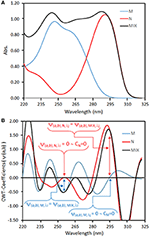Figure 2. (A) Absorption spectra and (B) CWT spectra of M () and N () compounds and their mixture ().

If CWT is applied to Equation (21), the following function can be obtained as

If ψ(a.b), N, λiCN = 0, then we obtain the following equation

Equation (23) shows that CWT (ψ(a.b), M, λi CM) amplitudes of M in the binary mixture are dependent only on CM regardless of CN (see Figure 2B).

## Ratio Spectra-Continuous Wavelet Transform

Apart from CWT-ZC approach, overlapping spectral bands in a binary mixture could be solved by the application of a combined hybrid approach i.e., RS-CWT (Dinç and Baleanu, 2004a,c).

The absorption spectra of M and N compounds, and their mixture are indicated in Figure 3A. By being divided by the standard spectrum () of one of the compounds in the binary mixture, Equation (21) becomes

Figure 3B shows the ratio spectra of analytes and their binary mixture. If CWT is applied to Equation (24), the following equation can be obtained

If = 0, then we obtain

The ratio-CWT amplitudes of the binary mixture given in Equation (26) depend only on CM and ${{\text{C}}_{\text{N}}}^{\text{o}}$ regardless of CN (e.g., see Figure 3C).

FIGURE 3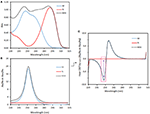Figure 3. (A) Absorption spectra, (B) ratio spectra, and (C) Haar CWT spectra of M and N compounds and their binary mixture.

## Ratio Spectra-Continuous Wavelet Transform-Zero Crossing

In RS-CWT-ZC approach (Dinç et al., 2005a), if a mixture of three analytes (X, Y, and Z) is considered and the absorbance of this ternary mixture is measured at λi, the following mathematical expression (Charlotte Grinter and Threlfall, 1992) would be given

Where Amix, λi is the absorbance of the ternary mixture at wavelength λi, and coefficients αX, λi, βY, λi, and γZ, λi denote the absorptivities of X, Y, and Z, respectively. CX, CY, and CZ represent the concentrations of X, Y, and Z, respectively.

If Equation (27) is divided by the spectrum of a standard solution (${\text{C}}_{\text{X}}^{\text{o}}$) of one of the compounds in the ternary mixture, we have the following equation:

If CWT is applied to Equation (28), the following equation can be obtained

Equation (29) indicates that the CWT amplitudes of the ratio spectra of the ternary mixture are dependent only on CZ and ${{\text{C}}_{\text{X}}}^{\text{o}}$ regardless of the concentrations of other compounds.

## Double Divisor Ratio Spectra-Continuous Wavelet Transform

In addition to RS-CWT-ZC approach, the spectral resolution of ternary mixtures could be effectively done by DDRS-CWT approach (Dinç and Baleanu, 2008a) as follows.

When two compounds in the ternary mixture is used as a double divisor, we have

By dividing Equation (27) and (30), we obtain as follows

Equation (31) can be simplified to

Where represents a constant for a given concentration range with respect to λi in a certain region or point of wavelength.

A typical case is when ${{\text{C}}_{\text{X}}}^{\text{o}}$ and ${{\text{C}}_{\text{Y}}}^{\text{o}}$ are the same or very close to each other, namely ${{\text{C}}_{\text{X}}}^{\text{o}}$ = ${{\text{C}}_{\text{Y}}}^{\text{o}}$ or $\cong {{\text{C}}_{\text{X}}}^{\text{o}}\cong {{\text{C}}_{\text{Y}}}^{\text{o}}$. Therefore, we obtain

and Equation (32) can be written as

After applying CWT to Equation (31), we have

or

In Equation (36), CZ is to proportional to the coefficients, , at λi. If this procedure is separately applied for pure Z and its ternary mixture, the CWT(a, b) coefficients are coincided at some characteristic point or region of wavelength, independent upon both CX and CY.

## Wavelet Transform-Based UV Spectroscopic Analysis of Pharmaceuticals

Typical applications of CWT and FWT algorithms for UV spectroscopic analysis of pharmaceuticals are displayed in Tables 25. It is worth mentioning that WT could be solely applied to raw spectra and ratio spectra (as above-specified) as well as utilized as a hybrid approach (FWT-derivative, FWT-CWT-zero crossing, WT combined with multivariate calibration) for the simultaneous determination of analytes in pharmaceutical binary and ternary mixtures. It was shown that wavelet analysis of UV spectroscopic data was performed by using Wavelet Toolbox and m-file in MATLAB software. The numerous works provided by Dinç and co-workers have clearly highlighted the success of WT-based UV spectroscopic analysis for multicomponent synthetic mixtures, veterinary and pharmaceutical dosage forms as well as different types of test (e.g., assay, in vitro dissolution, stability indicating). Most studies proved it to be suitable for the routine analysis of dosage forms with good precision and accuracy, comparable to HPLC.

TABLE 2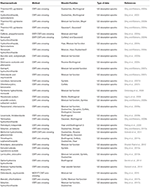Table 2. Applications of the continuous wavelet transform-zero crossing technique to UV spectroscopic analysis of pharmaceuticals.

TABLE 3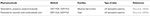Table 3. Applications of the wavelet transform-multivariate approaches to UV spectroscopic analysis of pharmaceuticals.

TABLE 4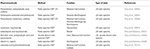Table 4. Applications of the ratio spectra-continuous wavelet transform, ratio spectra- continuous wavelet transform-zero crossing approaches to UV spectroscopic analysis of pharmaceuticals.

TABLE 5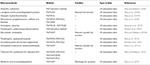Table 5. Applications of the fractional wavelet transform and its combination with other chemometric techniques to UV spectroscopic analysis of pharmaceuticals.

## Conclusions

In the point of view of UV spectroscopic analysis of multicomponent mixtures, CWT-based UV spectroscopic methods have outperformed both conventional and derivative UV spectroscopy in resolving spectrally binary and ternary mixtures. Nevertheless, wavelet analysis may not also have a sufficient power to resolve overlapping spectra of analytes in samples due to similarity of molecular structures and signal frequencies in some cases. They may not give desirable results for a complex mixture containing more than three compounds and/or a significant difference in ratios of active ingredients. In such a case, the use of WT coupled with chemometric PLS and PCR calibrations is advisable. Undoubtedly, however, wavelets can still be used as a mathematical prism for signal analysis because they can offer many possibilities such as baseline correction, noise removal and resolution of overlapping peaks, when the frequencies of analyzed components are significantly different from each other.

## Author Contributions

Contributions of ED are planning and writing of the review paper. Contributions of ZY are literature review, collection, editing, and format arrangement.

## Conflict of Interest Statement

The authors declare that the research was conducted in the absence of any commercial or financial relationships that could be construed as a potential conflict of interest.

## References

Aballe, A., Bethencourt, M., Botana, F. J., and Marcos, M. (1999). Using wavelet transform in the analysis of electrochemical noise data. Electrochim. Acta 44, 4805–4816. doi: 10.1016/S0013-4686(99)00222-4

Alsberg, B. K., Woodward, A. M., and Kell, D. B. (1997). An introduction to wavelet transform for chemometricians: a time-frequency approach. Chemom. Intell. Lab. Syst. 37, 215–239. doi: 10.1016/S0169-7439(97)00029-4

Barache, D., Antoine, J. P., and Dereppe, J. M. (1997). The continuous wavelet transform, an analysis tool for NMR spectroscopy. J. Magn. Reson. 128, 1–11. doi: 10.1006/jmre.1997.1214

Barclay, V. J., Bonner, R. F., and Hamilton, I. P. (1997). Application of wavelet transforms to experimental spectra: smoothing, denoising, and data set compression. Anal. Chem. 69, 78–90. doi: 10.1021/ac960638m

Berzas Nevado, J. J., Guiberteau Cabanillas, C., and Salinas, F. (1992). Spectrophotometric resolution of ternary mixtures of salicylaldehyde, 3-hydroxybenzaldehyde and 4-hydroxybenzaldehyde by the derivative ratio spectrum-zero crossing method. Talanta 39, 547–553. doi: 10.1016/0039-9140(92)80179-H

Blu, T., and Unser, M. (2000). “The fractional spline wavelet transform: definition and implementation,” in Proceedings of the Twenty-Fifth IEEE International Conference on Acoustics, Speech, and Signal Processing (ICASSP'00) (Istanbul), 512–515.

Blu, T., and Unser, M. (2002). Wavelets, fractals, and radial basis functions. IEEE Trans. Signal Process. 50, 543–553. doi: 10.1109/78.984733

Brereton, R. G. (2003). Data Analysis for the Laboratory and Chemical Plant. New York, NY: Wiley & Sons, Inc., 167.

Brereton, R. G. (2008). Applied Chemometrics for Scientists. John-Wiley & sons Ltd.

Brown, S., Blank, T. B., Sum, S. T., and Weyer, L. G. (1994). Chemometrics. Anal. Chem. 66, 315R−359R. doi: 10.1021/ac00084a014

Brown, S., Sum, S. T., and Despagne, F. (1996). Chemometrics. Anal. Chem. 68, 21R−62R. doi: 10.1021/a1960005x

Byrnes, J. S., Byrnes, J. L., Hargreaves, K. A., and Berry, K. (1994). Wavelet and Their Application. Netherlands: Kluwer Academic Publishers.

Cai, W. S., Wang, L. Y., Pan, Z. X., Zuo, J., Xu, C. Y., and Shao, X. G. (2001). Application of the wavelet transform method in quantitative analysis of raman spectra. J. Raman Spectrosc. 32, 207–209. doi: 10.1002/jrs.688

Çelebier, M., Altinöz, S., and Dinç, E. (2010). “Fractional wavelet transform and chemometric calibrations for the simultaneous determination of amlodipine and valsartan in their complex mixture,” in New Trends in Nanotechnology and Fractional Calculus, eds D. Baleanu, Z. B. Güvenç, and J. A. Tenreiro Machado (Dordrecht; Heidelberg; London; New York, NY: Springer), 333–340.

Chalus, P., Walter, S., and Ulmschneider, M. (2007). Combined wavelet transform–artificial neural network use in tablet active content determination by near-infrared spectroscopy. Anal. Chim. Acta 591, 219–224. doi: 10.1016/j.aca.2007.03.076

Charlotte Grinter, H., and Threlfall, T. L. (1992). UV-VIS Spectroscopy and its Applications. Berlin; Heidelberg; New York, NY: Springer-Verlag.

Chau, F., Yi-Zeng, L., Junbin, G., Xue-Guang, S., and James, D.W (2004). Chemometrics, from Basics to Wavelet Transform 1st Edn. Hoboken, NJ: Wiley-Interscience.

Chen, J., Zhong, H. B., Pan, Z. X., and Zhang, M. S. (1996). Application of the wavelet transform in differential pulse voltammetric data processing. Chin. J. Anal. Chem. 24, 1002–1006.

Chui, C. K. (1992). An Introduction to Wavelets. New York, NY: Academic Press. 49.

Chui, C. K., Montefusco, L., and Puccio, L. (1994). Wavelets: Theory, Algorithms and Applications. San Diego, CA: Academic Press.

Chun-Lin, L. (2010). A Tutorial of the Wavelet Transform. Available online at: http://disp.ee.ntu.edu.tw/tutorial/WaveletTutorial.pdf

Cooper, J. W. (1978). “Chapter: Data handling in fourier transform spectroscopy,” in Transform Techniques in Chemistry, ed P. R. Griffiths (New York, NY: Plenum Press), 4, 69–108.

Danzer, K. (2007). Analytical Chemistry. Theoretical and Metrological Fundamentals. Berlin; New York, NY: Springer.

Darwish, H. W., Metwally, F. H., and El.Bayoumi, A. (2014). Application of continous wavelet transform for derivative spectrophotometric determination of binary mixture in pharmaceutical dosage form. Dig. J. Nanomater. Biostruct. 9, 7–18.

Daubechies, I. (1992). Ten Lectures on Wavelets, Society for Industrial and Applied Mathematics. Philadelphia, PA.

Depczynski, U., Jetter, K., Molt, K., and Niemoller, A. (1997). The fast wavelet transform on compact intervals as a tool in chemometrics. I. Mathematical background. Chem. Intell. Lab. Syst. 39, 19–27. doi: 10.1016/S0169-7439(97)00068-3

Devrim, B., Dinç, E., and Bozkir, A. (2014). Fast determination of diphenhydramine hydrochloride in reconstituted table syrups by CWT, PLS and PCR methods. Acta Pol. Pharm. 71, 721–729.

Dinç, E. (1999). The spectrophotometric multicomponent analysis of a ternary mixture of ascorbic acid, acetylsalicylic acid and paracetamol by the double divisor-ratio spectra derivative and ratio spectra-zero crossing methods. Talanta 48, 1145–1157. doi: 10.1016/S0039-9140(98)00337-3

Dinç, E. (2013). Wavelet transforms and applications in drug analysis, FABAD J. Pharm. Sci. 38, 159–165.

Dinç, E., Arslan, F., and Baleanu, D. (2009a). Alternative approaches to the spectral quantitative resolution of two-component mixture by wavelet families. J. Chil. Chem. Soc. 54, 28–35. doi: 10.4067/S0717-97072009000100007

Dinç, E., and Baleanu, D. (2003a). Multidetermination of thiamine HCl and pyridoxine HCl in their mixture using continuous daubechies and biorthogonal wavelet analysis. Talanta 59, 707–717. doi: 10.1016/S0039-9140(02)00611-2

Dinç, E., and Baleanu, D. (2003b). A zero-crossing technique for the multidetermination of thiamine HCl and pyridoxine HCl in their mixture by using one-dimensional wavelet transform. J. Pharm. Biomed. Anal. 31, 969–978. doi: 10.1016/S0731-7085(02)00705-7

Dinç, E., and Baleanu, D. (2004a). Multicomponent quantitative resolution of binary mixtures using continuous wavelet transform. J. AOAC Int. 87, 360–365.

Dinç, E., and Baleanu, D. (2004b). Application of the wavelet method for the simultaneous quantitative determination of benazepril and hydrochlorothiazide in their mixtures. J. AOAC Int. 87, 834–841.

Dinç, E., and Baleanu, D. (2004c). One-dimension continuous wavelet resolution for the simultaneous analysis of binary mixture of benazepril and hydrochlorothiazide in tablets using spectrophotometric absorbance data. Rev. Roum. Chim. 49, 917–925.

Dinç, E., and Baleanu, D. (2006). A new fractional wavelet approach for the simultaneous determination of ampicillin sodium and sulbactam sodium in a binary mixture. Spectrochim. Acta Part A 63, 631–638. doi: 10.1016/j.saa.2005.06.012

Dinç, E., and Baleanu, D. (2007a). Continuous wavelet transform and chemometric methods for quantitative resolution of a binary mixture of quinapril and hydrochlorothiazide in tablets. J. Braz. Chem. Soc. 18, 962–968. doi: 10.1590/S0103-50532007000500013

Dinç, E., and Baleanu, D. (2007b). “A review on the wavelet transforms applications in analytical chemistry,” in Mathematical Methods in Engineering, eds K. Taş, J. A. Tenreiro Machado, and D. Balanu (Dordrecht: Springer), 265–285.

Dinç, E., and Baleanu, D. (2007c). Continuous wavelet transform applied to the overlapping absorption signals and their ratio signals for the quantitative resolution of mixture of oxfendazole and oxyclozanide in bolus. J. Food Drug Anal. 15, 109–117.

Dinç, E., and Baleanu, D. (2008a). Application of haar and mexican hat wavelets to double divisor-ratio Spectra for the multicomponent determination of ascorbic acid, acetylsalicylic acid and paracetamol in effervescent tablets. J. Braz. Chem. Soc. 19, 434–444. doi: 10.1590/S0103-50532008000300010

Dinç, E., and Baleanu, D. (2008b). Ratio spectra-continuous wavelet transform and ratio spectra-derivative spectrophotometry for the quantitative analysis of effervescent tablets of vitamin C and aspirin. Rev. Chim. (Bucuresti) 59, 499–504.

Dinç, E., and Baleanu, D. (2009a). Continuous wavelet transform applied to the quantitative analysis of a binary mixture. Rev. Chim. (Bucuresti) 60, 216–221.

Dinç, E., and Baleanu, D. (2009b). Spectral continuous wavelet transform for the simultaneous spectrophotometric analysis of a combined pharmaceutical formulation. Rev. Chim. 60, 741–744.

Dinç, E., and Baleanu, D. (2010a). Continuous wavelet transform applied to the simultaneous spectrophotometric determination of valsartan and amlodipine in tablets. Rev. Chim. 61, 290–294.

Dinç, E., and Baleanu, D. (2010b). Fractional wavelet transform for the quantitative spectral resolution of the composite signals of the active compounds in a two-component mixture. Comput. Math. Appl. 59, 1701–1708. doi: 10.1016/j.camwa.2009.08.012

Dinç, E., and Baleanu, D. (2012). Fractional-continuous wavelet transforms and ultra-performance liquid chromatography for the multicomponent analysis of a ternary mixture containing thiamine, pyridoxine, and lidocaine in ampules. J. AOAC Int. 95, 903–912. doi: 10.5740/jaoacint.11-199

Dinç, E., Baleanu, D., and Abaul-Enein, H. Y. (2004a). Wavelet analysis for the multicomponent determination in a binary mixture of caffeine and propyphenazone in tablets. IL Farmaco 59, 335–342. doi: 10.1016/j.farmac.2004.01.002

Dinç, E., Baleanu, D., Ioele, G., De Luca, M., and Ragno, G. (2008). Multivariate analysis of paracetamol, propyphenazone, caffeine and thiamine in quaternary mixtures by PCR, PLS and ANN calibrations applied on wavelet transform data. J. Pharm. Biomed. Anal. 48, 1471–1475. doi: 10.1016/j.jpba.2008.09.035

Dinç, E., Baleanu, D., and Kanbur, M. (2005c). A comparative application of wavelet approaches to the absorption and ratio spectra for the simultaneous determination of diminazene aceturate and phenazone in veterinary granules for injection. Pharmazie 60, 892–896.

Dinç, E., Baleanu, D., and Taş, A. (2006b). Wavelet transforms and artificial neural network for the quantitative resolution of ternary mixtures. Rev. Chim. 57, 626–631.

Dinç, E., Baleanu, D., and Taş, K. (2007a). “Continuous wavelet analysis for the ratio signals of the absorption spectra of binary mixtures,” in Mathematical Methods in Engineering, ed Taş, K., J. A. Tenreiro Machado, and D. Baleanu (Dordrecht: Springer), 285–293.

Dinç, E., Baleanu, D., and Taş, K. (2007b). Fractional wavelet analysis for the composite signals of two-component mixture by multivariate spectral calibration. J. Vib. Control 13, 1283–1290. doi: 10.1177/1077546307077464

Dinç, E., Baleanu, D., and Üstündag, Ö. (2003). An approach to quantitative two-component analysis of a mixture containing hydrochlorothiazide and spironolactone in tablets by one-dimensional continuous daubechies and biorthogonal wavelet analysis of UV-spectra, Spectros. Lett. 36, 341–355. doi: 10.1081/SL-120024583

Dinç, E., Baleanu, D., Üstündag, Ö., and Abaul-Enein, H. Y. (2004c). Continuous wavelet transformation applied to the simultaneous quantitative analysis of two–component mixtures. Pharmazie 59, 618–623.

Dinç, E., Büker, E., and Baleanu, D. (2011a). Fractional and continuous wavelet transforms for the simultaneous spectral analysis of a binary mixture system. Commun. Nonlinear Sci. Numer. Simul. 16, 4602–4609. doi: 10.1016/j.cnsns.2011.02.018

Dinç, E., Demirkaya, F., Baleanu, D., Kadioglu, Y., and Kadioglu, E. (2010). New approach for simultaneous spectral analysis of a complex mixture using the fractional wavelet transform. Commun. Nonlinear Sci. Numer. Simul. 15, 812–818. doi: 10.1016/j.cnsns.2009.05.021

Dinç, E., Duarte, F. B., Tenreiro Machado, J. A., and Baleanu, D. (2015). Application of continuous wavelet transform to the analysis of the modulus of the fractional fourier transform bands for resolving two component mixture. Signal Image Video P. 9, 801–807. doi: 10.1007/s11760-013-0503-9

Dinç, E., Kadioglu, Y., Demirkaya, F., and Baleanu, D. (2011b). Continuous wavelet transforms for simultaneous spectral determination of trimethoprim and sulphamethoxazole in tablets. J. Iran. Chem. Soc. 8, 90–99. doi: 10.1007/BF03246205

Dinç, E., Kanbur, M., and Baleanu, D. (2007c). Comparative spectral analysis of veterinary powder product by continuous wavelet and derivative transforms. Spectrochim. Acta Part A. 68, 225–230. doi: 10.1016/j.saa.2006.11.018

Dinç, E., Kaş, F., and Baleanu, D. (2013a). A signal processing tool based on the continuous wavelet for the simultaneous determination of estradiol valerate and cyproterone acetate in their mixtures. Rev. Chim. 64, 124–126.

Dinç, E., Kaya, S., Doganay, D., and Baleanu, D. (2007d). Continuous wavelet and derivative transforms for the simultaneous quantitative analysis and dissolution test of levodopa-benserazide tablets. J. Pharm. Biomed. Anal. 44, 991–995. doi: 10.1016/j.jpba.2007.03.027

Dinç, E., and Onur, F. (1998). Application of a new spectrophotometric method for the analysis of a ternary mixture containing metamizol, paracetamol and caffeine in tablets. Anal. Chim. Acta 359, 93–106. doi: 10.1016/S0003-2670(97)00615-6

Dinç, E., Özdemir, A., and Baleanu, D. (2005a). An application of derivative and continuous wavelet transforms to the overlapping ratio spectra for the quantitative multiresolution of a ternary mixture of paracetamol, acetylsalicylic acid and caffeine in tablets. Talanta 65, 36–47. doi: 10.1016/j.talanta.2004.05.011

Dinç, E., Özdemir, A., and Baleanu, D. (2005b). Comparative study of the continuous wavelet transform, derivative and partial least squares methods applied to the overlapping spectra for the simultaneous quantitative resolution of ascorbic acid and acetylsalicylic acid in effervescent tablets. J. Pharm. Biomed. Anal. 37, 569–575. doi: 10.1016/j.jpba.2004.11.020

Dinç, E., Özdemir, A., Baleanu, D., and Taş, K. (2006a). Wavelet transform with chemometrics techniques for quantitative multiresolution analysis of a ternary mixture consisting of paracetamol, ascorbic acid and acetylsalicylic acid in effervescent tablets. Rev. Chim. (Bucharest) 57, 505–510.

Dinç, E., Özdemir, N., Üstündag, Ö., and Günseli Tilkan, M. (2013b). Continuous wavelet transforms for the simultaneous quantitative analysis and dissolution testing of lamivudine–zidovudine tablets. Chem. Pharm. Bull. 61, 1220–1227. doi: 10.1248/cpb.c13-00284

Dinç, E., Pektaş, G., and Baleanu, D. (2009b). Continuous wavelet transform and derivative spectrophotometry for the quantitative spectral resolution of a mixture containing levamizole and triclabendazole in veterinary tablets. Rev. Anal. Chem. 28, 79–92. doi: 10.1515/REVAC.2009.28.2.79

Dinç, E., Ragno, G., Baleanu, D., De Luca, M., and Ioele, G. (2012). Fractional wavelet transform-continuous wavelet transform for the quantification of melatonin and its photodegradation product. Spectrosc. Lett. 45, 337–343. doi: 10.1080/00387010.2012.666699

Dinç, E., Ragno, G., Ioele, G., and Baleanu, D. (2006c). Fractional wavelet analysis for the simultaneous quantitative analysis of lacidipine and its photodegradation product by continuous wavelet transform and multilinear regression calibration. JAOAC Int. 89, 1538–1546.

Dinç, E., Saygeçitli, E., and Ertekin, Z. C. (2017b). Simultaneous determination of atenolol and chlorthalidone in tablets by wavelet transform methods. FABAD J. Pharm. Sci. 42, 103–109.

Dinç, E., Üstündaǧ, Ö., Yüksel Tilkan, G., Türkmen, B., and Özdemir, N. (2017a). Continuous wavelet transform methods for the simultaneous determinations and dissolution profiles of valsartan and hydrochlorothiazide in tablets. Braz. J. Pharm. Sci. 53:e16050. doi: 10.1590/s2175-97902017000116050

Dinç, E., Baleanu, D., and Kanbur, M. (2004b). Spectrophotometric multicomponent determination of tetramethrin, propoxur and piperonyl butoxide in insecticide formulation by principal component regression and partial least squares techniques with continuous wavelet transform. Can. J. Anal. Sci. Spect. 49, 218–225.

Dubrovkin, J. (2018). Mathematical Processing of Spectral Data in Analytical Chemistry: A Guide to Error Analysis. Newcastle upon Tyne: Cambridge Scholars Publishing.

Ehrentreich, F., and Summchen, L. (2001). Spike removal and denoising of Raman spectra by wavelet transform methods. Anal. Chem. 73, 4364–4373. doi: 10.1021/ac0013756

Ernst, R. R. (1989). Nuclear magnetic resonance fourier transform spectroscopy. Bull. Magn. Resonance 16, 5–29.

Fang, H., and Chen, H. Y. (1997). Wavelet analyses of electroanalytical chemistry responses and an adaptive wavelet filter. Anal. Chim. Acta 346, 319-−325. doi: 10.1016/S0003-2670(97)90071-4

Gohel, R. V., Parmar, S. J., and Patel, B. A. (2014). Development and validation of double divisor-ratio spectra derivative spectrophotometric method for simultaneous estimation of olmesartan medoxomil, amlodipine besylate and hydrochlorthiazide in tablet dosage form. Int. J. Pharm.Tech. Res. 6, 1518–1525.

Griffiths, P. R. (1977). Recent applications of fourier transform infrared spectrometry in chemical and environmental analysis. Appl. Spectros. 31, 497–505. doi: 10.1366/000370277774464084

Griffiths, P. R., and De Haseth, J. A. (1986). Fourier Transform Infrared Spectroscopy. New York, NY: Wiley.

Hwang, M. S., Choa, C., Chunga, H., and Woo, Y. A. (2005). Nondestructive determination of the ambroxol content in tablets by Raman spectroscopy. J. Pharm. Biomed. Anal. 38, 210–215. doi: 10.1016/j.jpba.2004.12.031

Jun-fang, X., Xiao-yu, L., Pei-wu, L., Qian, M. A., and Xiao-xia, D. (2007). Application of wavelet transform in the prediction of navel orange vitamin C content by near-infrared spectroscopy. Agr. Sci. China 6, 1067–1073. doi: 10.1016/S1671-2927(07)60148-5

CrossRef Full Text

Kambur, M., Narin, I., Dinç, E., Candir, S., and Baleanu, D. (2011). Fractional wavelet transform for the quantitative spectral resolution of the commercial veterinary preparations. Rev. Chim. (Bucuresti) 62, 618–621.

Kanbur, M., Narin, I., Özdemir, E., and Dinç, E. (2010). “Fractional wavelet transform for the quantitative spectral analysis of two-component system,” New Trends in Nanotechnology and Fractional Calculus, eds D. Baleanu, Z. B. Güvenç, and J. A. Tenreiro Machado (Dordrecht; Heidelberg; London; New York, NY: Springer), 321–331.

Lai, Y., Nia, N., and Kokot, S. (2011). Discrimination of Rhizoma Corydalis from two sources by near-infrared spectroscopy supported by the wavelet transform and least-squares support vector machine methods. Vib. Spectrosc. 56, 154–160. doi: 10.1016/j.vibspec.2011.01.007

Leung, A. K. M., Chau, F.-T., and Gau, J.-B. (1998). A review on applications of wavelets transform techniques in chemical analysis, 1989-1997. Chem. Intell. Lab. Sys. 43, 165–184. doi: 10.1016/S0169-7439(98)00080-X

Levillain, P., and Fompeydie, D. (1986). Spectrophotométrie dérivée: intérêt, limites et applications. Analusis 14, 1–20.

Li, B., and Chen, X. (2014). Wavelet-based numerical analysis: a review and classification. Finite Elem. Anal. Des., 81, 14–31. doi: 10.1016/j.finel.2013.11.001

Mallat, S. (1988). A Wavelet Tour of Signal Processing. New York, NY: Academic Press.

Mark, H., and Workman, J. (2007). Chemometrics in Spectroscopy. London; San Diego, CA; Cambridge, MA, Oxford: Elsevier.

Medhat, M. E. (2015). A review on applications of the wavelet transform technique in spectral analysis. J. Appl. Computat. Math. 4, 1–6. doi: 10.4172/2168-9679.1000224

Neue, G. (1996). Simplification of dynamic NMR spectroscopy by wavelet transform. Solid State Nucl. Magn. Reson. 5, 305–314.

Newland, D. E. (1993). An Introduction to Random Vibrations, Spectral and Wavelet Analysis. Longman: University of Cambridge. 295–370.

O'Haver, T. C. (1979). Derivative and wavelength modulation spectrometry. Anal. Chem. 51, 91A−100A.

O'Haver, T. C., and Green, G. L. (1976). Numerical error analysis of derivative spectrometry for the quantitative analysis of mixtures. Anal. Chem. 48, 312–318.

Palavajjhala, S., Motard, R. L., and Joseph, B. (1994). Wavelet Application in Chemical Engineering. eds R. L. Motard and B. Joseph (Norwell, MA: Kluwer Academic Publishers), 33–83.

Pektaş, G., Dinç, E., and Baleanu, D. (2009). Combined application of continuous wavelet transform-zero crossing technique in the simultaneous spectrophotometric determination of perindopril and indapamid in tablets. Quím. Nova 32, 1416–1421.

Ragno, G., Ioele, G., De Luca, M., Garofalo, A., Grande, F., and Risoli, A. (2006). A critical study on the application of the zero-crossing derivative spectrophotometry to the photodegradation monitoring of lacidipine. J. Pharm. Biom. Anal. 42, 39–45. doi: 10.1016/j.jpba.2005.11.025

Salinas, F., Berzas Nevado, J. J., and Espinoso Mansilla, A. E. (1990). A new spectrophotometric method for quantitative multicomponent analysis resolution of mixtures of salicylic and salicyluric acids. Talanta 37, 347–351. doi: 10.1016/0039-9140(90)80065-N

Shao, X., Cai, W., and Sun, P. (1998a). Determination of the component number in overlapping multicomponent chromatogram using wavelet transform. Chemom. Intell. Lab. Syst. 43, 147–155.

Shao, X., Cai, W., Sun, P., Zhang, M., and Zhao, G. (1997). Quantitative Determination of the components in overlapping chromatographic peaks using wavelet transform. Anal. Chem. 69, 1722–1725. doi: 10.1021/ac9608679

Shao, X., Hou, S., Fang, N., He, Y., and Zhao, G. (1998b). Quantitative determination of plant hormones by high performance liquid chromatography with wavelet transform. Chin. J. Anal. Chem. 26, 107–110.

Shao, X., Hou, S., and Zhao, G. (1998c). Extraction of the component information from overlapping chromatograms by wavelet transform. Chin. J. Anal. Chem. 26, 1428–1431.

Shao, X., and Zhuang, Y. (2004). Determination of chlorogenic acid in plant samples by using near-infrared spectrum with wavelet transform preprocessing. Anal. Sci. 20, 451–454. doi: 10.2116/analsci.20.451

Shariati-Rad, M., Irandoust, M., Amini, T., and Ahmadi, F. (2012). Partial least squares and continuous wavelet transformation in simultaneous spectrophotometric determination of amlodipin and atorvastatine. Pharm. Anal. Acta 3:178. doi: 10.4172/2153-2435.1000178

Shokry, E., El-Gendy, A. E., Kawy, M. A., and Hegazy, M. (2014). Application of double divisor ratio spectra derivative spectrophotometric [DDRS-DS], chemometric and chromatographic methods for stability indicating determination of moexipril hydrochloride and hydrochlorothiazide. Curr. Sci. Int. 3, 352–380.

Sohrabi, M. R., Kamali, N., and Khakpour, M. (2011). Simultaneous spectrophotometric determination of metformin hydrochloride and glibenclamide in binary mixtures using combined discrete and continuous wavelet transforms. Anal. Sci. 27, 1037–1041.

Strang, G., and Nguyen, T.(eds.). (1996). Wavelets and Filter Banks. MA: Wellesley-Cambridge Press. 72.

Ugurlu, G., Özaltin, N., and Dinç, E. (2008). Spectrophotometric determination of risedronate sodium in pharmaceutical preparations by derivative and continuous wavelet transforms. Rev. Anal. Chem. 27, 215–233. doi: 10.1515/REVAC.2008.27.4.215

Unser, M., and Blu, T. (2000). Fractional splines and wavelets. SIAM Rev. 42, 43–67. doi: 10.1137/S0036144598349435

Üstündag, Ö., Dinç, E., and Baleanu, D. (2008). Applications of Mexican hat wavelet function to binary mixture analysis. Rev. Chim. (Bucuresti) 59, 1387–1391.

Vetterli, M., and Kovačević, J. (1995). Wavelets and Subband Coding. Englewood Cliffs, NJ: Prentice Hall PTR.

Walczak, B., and Massart, D. (1997a). Wavelet packet transform applied to a set of signals: a new approach to the best-basis selection. Chemom. Intell. Lab. Syst. 38, 39–50. doi: 10.1016/S0169-7439(97)00050-6

Walczak, B., and Massart, D. L. (1997b). Noise suppression and signal compression using the wavelet packet transform. Chemom. Intell. Lab. Sys. 36, 81–94. doi: 10.1016/S0169-7439(96)00077-9

Walczak, B., and Massart, D. L. (1997c). Wavelets-something for analytical chemistry. Trends Analyt. Chem. 16, 451–463. doi: 10.1016/S0165-9936(97)00065-4

Walczak, B., and Massart, D. L. (2000a). “Calibration in wavelet domain,” in Wavelets in Chemistry, ed B. Walczak (Amsterdam: Elsevier), 323–347.

Walczak, B., and Massart, D. L. (2000b). “Joint basis and joint best-basis for data sets,” in Wavelets in Chemistry, ed B. Walczak (Amsterdam: Elsevier), 165–171.

Walczak, B., and Radomski, J. P. (2000). “Wavelet bases for IR library compression, searching and reconstruction,” in Wavelets in Chemistry, ed B. Walczak (Amsterdam: Elsevier), 291–308.

Wang, H., Xiao, J., Fan, Z., and Zhang, M. (1997). Wavelet transform and its application in chemistry. Chem. Bull. Huaxue Tongbao 6, 20–23.

Wickerhauser, M. V. (1994). Adapted Wavelet Analysis From Theory to Software. New York, NY:A.K. Peters, ltd.

Yan-Fang, S. (2013). A review on the applications of wavelet transform in hydrology time series analysis. Atmospheric Res.122, 8–15. doi: 10.1016/j.atmosres.2012.11.003

Zheng, X. P., and Mo, J. Y. (1999). The coupled application of the B-spline wavelet and RLT filtration in staircase voltammetry. Chemometr. Intell. Lab. Syst. 45, 157-−161. doi: 10.1016/S0169-7439(98)00099-9

Zheng, X. P., Mo, J. Y., and Cai, ‘P. X. (1998). Simultaneous application of spline wavelet and Riemann-Liouville transform filtration in electroanalytical chemistry. Anal. Commun. 35, 57–59.

Zhong, H. B., Zheng, J. B., Pan, Z. X., Zhang, M. S., and Gao, H. (1998). Investigation on application of wavelet transform in recovering useful information from oscillographic signal. Chem. J. Chin. Univ. 19, 547–549.

Keywords: discrete wavelet transform, continuous wavelet transform, fractional wavelet transform, UV spectroscopy, pharmaceutical analysis

Citation: Dinç E and Yazan Z (2018) Wavelet Transform-Based UV Spectroscopy for Pharmaceutical Analysis. Front. Chem. 6:503. doi: 10.3389/fchem.2018.00503

Received: 27 April 2018; Accepted: 03 October 2018;
Published: 26 October 2018.

Edited by:

Hoang Vu Dang, Hanoi University of Pharmacy, Vietnam

Reviewed by:

Gaetano Ragno, Università della Calabria, Italy
Joseph Dubrovkin, Western Galilee College, Israel

Copyright © 2018 Dinç and Yazan. This is an open-access article distributed under the terms of the Creative Commons Attribution License (CC BY). The use, distribution or reproduction in other forums is permitted, provided the original author(s) and the copyright owner(s) are credited and that the original publication in this journal is cited, in accordance with accepted academic practice. No use, distribution or reproduction is permitted which does not comply with these terms.

*Correspondence: Erdal Dinç, dinc@ankara.edu.tr